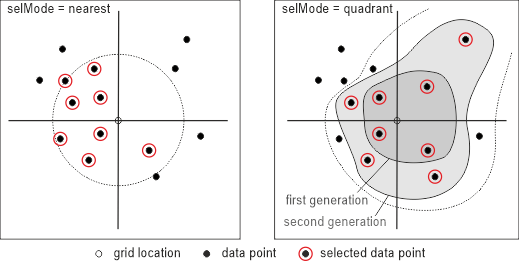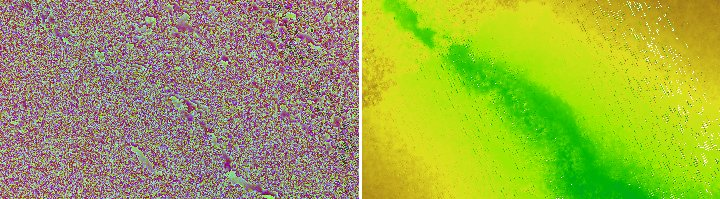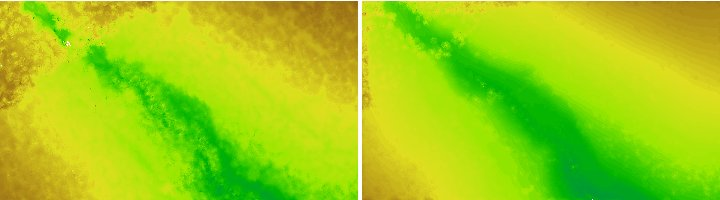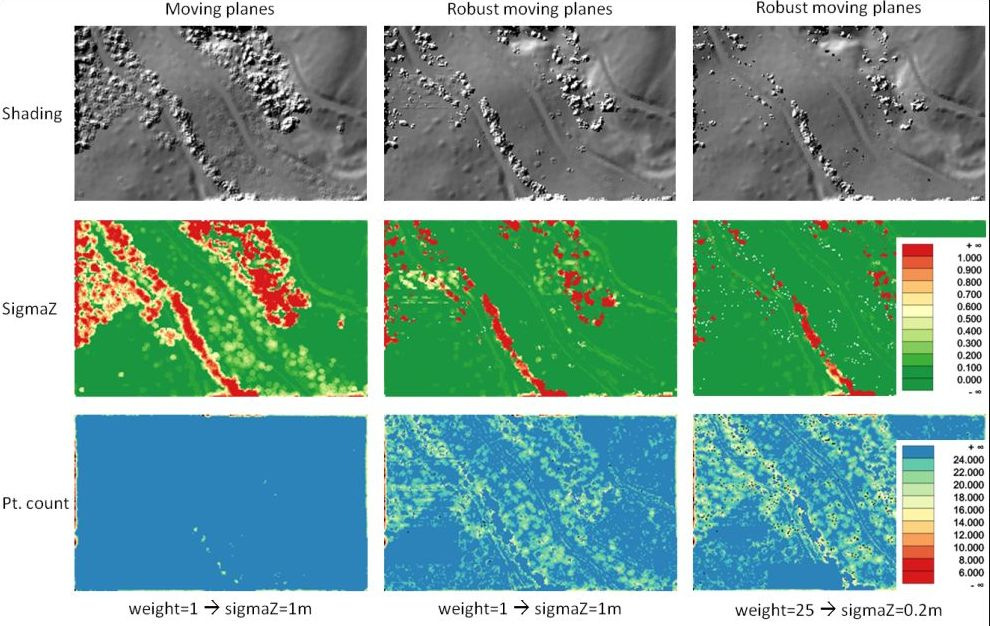Module Grid

opals::IGrid

# Aim of module

Derives a 2.5D digital elevation models (DEM) in regular grid structure using simple interpolation techniques.

# General description

Digital Surface Models (DSM) and Digital Terrain Models (DTM) are among the most important products derived from ALS point cloud data. DTM production based on ALS data is a complex task comprising (semi-)automatic modelling of structure lines, classifying the point cloud in terrain and off-terrain points and, finally, interpolating a smooth surface using high-level interpolation techniques like linear prediction or Kriging, respectively. In contrast, there are also a series of tasks within the ALS processing chain, which do not require the utmost quality concerning the geomorphological surface quality but, instead, shortest possible derivation times are crucial. This becomes especially true for the quality check of overlapping ALS strips (relative strip differences) based on distinct strip DSMs.

For this reason, Module Grid aims at deriving surface models in regular grid structure with maximum speed. To achieve high computation performance:

• the OPALS Data Manager (ODM) providing high performance nearest neighbour queries within the ALS point cloud is used as data basis
• multi-core CPUs of modern computers are used extensively
• only simple interpolation methods are provided
• only rectangular grids (rasters) are derived, i.e. no break lines are considered

The ODM serves as data basis (parameter inFile) for the grid interpolation. Thus, the import of ALS point cloud data, stored in arbitrary data formats, is a prerequisite for Module Grid. For details concerning data import, please refer to Module Import. The interpolation results are stored as a regular grid file (parameter outFile) in a GDAL supported data format (parameter outformat). The internal processing is organised in patches (tiles), thus, maximum performance can be achieved for data formats supporting tile wise storage like, GeoTiff or SCOP.RDH. Sequential data formats (e.g. ArcInfo ASCII grid, DTED ...) can else be written directly by Module Grid, but probably with lower performance. The derived grid model is strictly stored in "pixel is point" interpretation, i.e. the grid values represent the interpolated heights at the pixel center. However, user defined limits (parameter limits) for the output grid model can either refer to the centers (=default) or corners of the outermost pixels (c.f. parameter limit description). For a more detailed discussion "grid versus raster" please refer to the glossary.

The following interpolation strategies (parameter interpolation) ordered by increasing processing time are offered:

• Snap grid: This is the simplest method: For each point the appropriate grid cell is determined and the height of the point is mapped to this cell. Please note that subsequent ALS points belonging to the same grid cell overwrite previous values. The snap grid parameter is mainly useful in case of already gridded input points in order to avoid additional interpolation.
• Nearest neighbour: For each grid cell the nearest data point is queried from the ODM and the height of this nearest neighbour point is mapped to the grid cell. The quality of the derived surface is still poor, but computation performance is very high.
• Delaunay triangulation: The input points are triangulated and the grid heights are interpolated from the TIN. Please note, that the processing is tile based; for each tile a local TIN is constructed. To avoid data gaps additional points from neighbouring tiles are considered within a distance controlled by the parameter searchRadius.
• Moving average: For each grid cell n nearest points (parameter neighbours) are queried and the average height of all n points is mapped to the grid cell.
• Moving planes: For each grid cell n nearest points (parameter neighbours) are queried and a best fitting tilted plane (minimizing the vertical distances) is estimated. The height of the resulting plane at the grid point (x,y) position is mapped to the grid cell. The tilted plane interpolator allows the derivation of slope measures (nx, ny, slope, exposition) for each grid point.
• Robust moving planes: As above, with the difference that outliers are detected during the plane fitting using a robust estimation approach. Point classified as outliers do not take part of the plane fitting. To achieve reasonable results a high number of neighbouring points should be considered and an appropriate weight function should be provided
• Moving paraboloid: For each grid cell n nearest ALS points (parameter neighbours) are queried and a best fitting paraboloid (2nd order polynomial) is estimated. The height of the resulting paraboloid at the grid point (x,y,) position is mapped to the grid cell. The paraboloid interpolator allows the derivation of curvature measures (kmin,kmax,kmean,kgauss) for each grid point.

The last four methods (moving average, moving planes, moving paraboloid, robust moving planes) require the specification of the number of neighbouring points considered for the interpolation of a single grid post (parameter neighbours). The results of the neighbour queries can be restricted to a maximum search radius around the grid point (parameter searchRadius), thus, enabling to reflect areas with sparse point density in the resulting grid as void pixels.

## Data selection strategiesFig 1: Point data selection (parameter neighbours 8) in nearest mode (left) and quadrant mode (right)

As mentioned above, the primary aim of Module Grid is the derivation of surface grid models. In addition, additional feature models (parameter feature) can be derived simultaneously as some of them are side products of the grid interpolation and, thus, can be provided without loss of performance. The following additional features are supported:

• sigmaz: std.dev. of interpolated grid height (moving average/planes only)
• sigma0: std.dev. of the unit weight observation (moving average/planes only)
• density: point density estimate (moving average/planes only)
• excentricity: distance grid point - center of gravity of data points
• slope: steepest slope in % (moving planes only)
• slpDeg: steepest slope in degree (moving planes only)
• slope: steepest slope in % (moving planes only)
• exposition: slope aspect [rad] = azimuth of steepest slope line, N=0, clockwise sense of rotation (moving planes only)
• normalx: x-component of the surface normal unit vector (moving average/planes only)
• normaly: y-component of the surface normal unit vector (moving average/planes only)
• kmin: minimum curvature (moving paraboloid only)
• kmax: minimum curvature (moving paraboloid only)
• kmean: mean curvature H: H = (kmin+kmax)/2 (moving paraboloid only)
• kgauss: gaussian curvature K: kmin*kmax (moving paraboloid only)

By default, the additional features are stored as separate grid model files in identical grid structure and data format as the corresponding surface model. The file names are either constructed automatically, if only the name of the surface grid model file is defined, based on the definition of the respective global postfix parameters (postfix_sigma, postfix_pdens, postfix_excen, postfix_slope, postfix_slpDeg, postfix_slpRad, postfix_expos, postfix_nx, postfix_ny, postfix_kmin, postfix_kmax, postfix_kmean, postfix_kgauss,), or individual output file names can be specified. In the latter case, the number of entered file names must correspond to the number of derived grid models (surface model + feature models). Additional feature models are especially useful for subsequent creation of grid masks using Module Algebra. If the boolean parameter multiband is set to `true` or `1`, respectively, only a single raster file with multiple bands is created. The first band always contains the z/attribute-raster followed by the feature bands in the order specified by the user.

In general, each point of the point cloud has the same influence on the run of surface. However, sometimes it might be useful to give preference to certain points (e.g. points with higher amplitude/reflectance or points closer to the grid point). Individual point weights can be achieved by specifying a point weight function formula (weightFunc) as a string in generic filter syntax. Individual point weights are reasonable/supported for all k-nn based interpolators (e.g.: movingAverage, movingPlanes, movingParaboloid, robMovingmovingPlanes). The formula is evaluated for each of the k-nn points and must return a value which is used as weight for the respective point observation. To define a point weight, the following elements can be used:

• All attributes of the k-nn point (e.g., Amplitude, EchoWidth, etc.)
• The 2D-distance between the grid point and the knn-point. Within the formula, the grid point can be accessed as n and the k-nn point as n. To calculate the (squared) distance, the built-in functions SqrDist2D(n,n) and Dist2D(n,n). Please note, that only 2D distances can be used as the grid points do not contain height information (before the interpolation).

As a general rule, the formulas should be defined so that valid weights can be computed for each k-nn point. A grid point will automatically be set to void (no data) if the weight function cannot be calculated for a single point of the k-nn set. The most frequent causes for numeric problems are divisions by zero (e.g. distance in the denominator) which can often be avoided by using a proper epsilon. For Inverse Distance Weighing, for instance, predefined formulae are available (idw1, idw2, idw:n, c.f. parameter description below for details).

Please note, that the outlier classification procedure within the moving planes estimation relies on the individual point weights and, thus, the rejection level can be controlled by assigning global or individual point weights. It is good practice to use the height variance (varZ=sqr(sigmaZ)) of a point as the basis for the weight calculation, with w = 1 / varZ.

# Parameter description

-inFileinput data manager file name(s)
Type: opals::Vector<opals::Path>
Remarks: mandatory
The path(s) to the opals datamanager(s) whose point data are used to derive the surface grid model. In case multiple ODMs are specified, the data are queried from all (potentially overlapping) ODMs.
-outFileoutput gridfile name(s)
Type: opals::Vector<opals::Path>
Remarks: estimable
Path of output grid file in GDAL supported format.
Estimation rule: The current directory and the name (body) of the input file are used as file name basis. Additionally, the default extension of the output format is appended.
-oFormatgrid file format [GTiff,AAIGrid,USGSDEM,SCOP...]
Type: opals::String
Remarks: estimable
Use GDAL driver names like GTiff, AAIGrid, USGSDEM, SCOP... .
Estimation rule: The output format is estimated based on the extension of the output file (*.tif->GTiff, *.dem->USGSDEM, *.dtm->SCOP...).
-tileSizetile (block) size
Type: unsigned int
Remarks: default=64
The internal processing is organized in rectangular tiles. The size of these computing units(number of grid lines per tile) can be specified allowing control of the memory consumption of the module. Recommended values are in the range of 64 (default) if the average point distance approximately matches the grid size. In case coarser of finer grids are to be derived, the tile size may need to be adapted. Finer grids allow for larger tile sizes (and vice versa).
-gridSizegrid width x/y
Type: double
Remarks: default=1
Size of the rectangular grid cell in x- and y-direction. If only one value is passed, quadratic cells are used.
-attributeattribute for which a grid model shall be derived
Type: opals::String
Remarks: default=z
Attribute examples: z(default), Amplitude, EchoWidth, etc.
-interpolationinterpolation method
Type: opals::GridInterpolator
Remarks: default=snap

```Possible values:
snap .................... simple 'snap-height-of-nearest-data-point' interpolation
nearestNeighbour ........ nearest neighbour interpolation
delaunayTriangulation ... Delaunay triangulation interpolation
movingAverage ........... moving average interpolation (=moving horizontal plane)
movingPlanes ............ moving (tilted) plane interpolation
robMovingPlanes ......... robust moving (tilted) plane interpolation
movingParaboloid ........ moving circular, elliptic or hyperbolic paraboloid
kriging ................. kriging interpolation
```

The grid interpolation methods are ordered by quality. Higher-quality surfaces can be achieved using the latter methods but, in turn, the processing performance decreases.

-neighboursnr of nearest neighbours used for grid point interpolation
Type: int
Remarks: default=8
This parameter is only considered for moving average, planes and parabola interpolation.
Type: double
Remarks: estimable
Only points within the given search radius are considered for the interpolation of a single grid post. If the search area contains too few points for successful interpolation, the respective grid point is omitted (void).Estimation rule: searchRadius = 3 * gridSize .
-selModedata selection mode
Type: opals::SelectionMode
Remarks: default=nearest

```Possible values:
nearest .... pure nearest neighbour (nn) selection
```

Please note that quadrant wise data selection on the one hand reduces extrapolation effects, but on the other hand decreases the processing performance

-weightFuncpoint weight formula
Type: opals::String
Remarks: optional
An algebraic formula in generic filter syntax can be specified to calculate an individual weight for each point. Both, the points' attributes and the distance w.r.t the grid location can be used to determine the point weight. Inverse Distance Weighting (IDW) can be realized by using the built-in "Dist2D(n,n)" or "SqrDist2D(n,n)" function returning the linear or squared distance between the current grid location n and the considered neighbour point n. The following predefined formulae can be used :
IDW1.... inverse linear distance (i.e., 1/(1+ad))
IDW2.... inverse squared distance (i.e., 1/(1+(ad)^2)
IDW:i... inverse distance to the power of i ( i.e., 1/1+(ad)^i)
where 'd' denotes the 2D distance between the grid point and the neighbouring data point and 'a' the shape factor of the weight function. 'a' determines how fast the weight function drops to zero with increasing distance from the grid point. It is determined automatically in a way that points located at the border of the search circle (c.f. -searchRadius) obtain a small weight w=0.05.
-featureadditional output of feature grid models
Type: opals::Vector<opals::GridFeature>
Remarks: optional

```Possible values:
sigmaz ......... sigma z of grid post adjustment (i.e. std.dev. of the interpolated height)
sigma0 ......... sigma 0 of grid post adjustment (i.e. std.dev. of the unit weight observation)
pdens .......... point density estimate
pcount ......... number of points used for interpolation of grid point
excentricity ... distance between grid points - c.o.g. of data points
slope .......... steepest slope [%]
slpDeg ......... steepest slope [deg]
exposition ..... slope aspect [rad] (azimuth of steepest slope line, N=0, clockwise)
normalx ........ x-component of surface normal unit vector
normaly ........ y-component of surface normal unit vector
kmin ........... minimum curvature
kmax ........... maximum curvature
kmean .......... mean curvature: kmean=(kmin+kmax)/2
kgauss ......... gaussian curvature: kgauss = kmin*kmax
kminDir ........ Azimuth [rad] of minimum curvature
kmaxDir ........ Azimuth [rad] of maximum curvature
absKmaxDir ..... Azimuth [rad] of maximum absolute curvature
precision ...... grid post precision (derived from sigmaz, pdens and slope)
```

If one or more features are specified, additional grid models in the same format and structure as the basic surface model are derived. Please note that if more than one output file is specified, the number of output file names must match the number of specified features: nr. outFile = nr. feat. + 1

-filtermodify the input using a (tree of) filter(s)
Type: opals::String
Remarks: optional
A filter string in EBNF syntax as described in section 'Filter syntax' can be passed to restrict the set of input points (e.g. to consider last echoes only).
-limit2D clipping window
Type: opals::GridLimit
Remarks: optional
If no user defined limits are specified or -limit is even skipped, the OPALS Data Manager (ODM) extents are used. Rounding is enabled by default in case the limits are derived from the ODM and can be enforced for user defined limits (keyword: round).
-multiBandif multiband set to true then multiple bands will be used instead of multiple files.
Type: bool
Remarks: default=0
When using multiband grid features will be stored in multiple bands instead of multiple files.Features will be saved in the same order as user input.For example if -feature sigma0 slope then Band1=sigma0 and Band2=slope
-extrapolationCheckavoid extrapolation flag
Type: bool
Remarks: default=1
Unfavorable point distributions can lead to extrapolations, where the interpolated value clearly exceeds the local data values. Using the local data range extended by a buffer factory, such extrapolation are detected and rejected if this option is enabled.

# Short examples

## Generate a DEM from a LAS file

opalsImport -inf G111.las
opalsGrid -inf G111.odm
from opals.Import import Import
from opals.Grid import Grid
Import(inFile='G111.las').run()
Grid(inFile='G111.odm').run()

# Examples

The data used in the following examples can be found in the `\$OPALS_ROOT/demo/` directory. As the derivation of (surface) grid model relies on the ODM, the respective point cloud data has to be imported. The datasets `fullwave.fwf` and `fullwave2.fwf` containing full waveform ALS point data (x, y, z, gps time, amplitude, echo width, echo nr, echo qualifier) of a wooded area are used. The following pre-processing steps are required:

opalsImport -inf fullwave.fwf -iFormat FWF -coord_ref_sys EPSG:31256
opalsImport -inf fullwave2.fwf -outFile fwf.odm -iFormat FWF -coord_ref_sys EPSG:31256

## Example 1:

In the first example only the mandatory parameter (inFile) is specified. Thus, the command is very short and simple:

opalsGrid -inf fullwave.odm

It produces the grid file `fullwave_z.tif` in the default GeoTiff file format. The grid extensions are determined from the bounding box of the entire ODM point data set. The simple snap grid interpolation is used, thus a poor quality surface is derived. The grid size was not specified and, therefore, set to 1m.

## Example 2:

In the second example some of the optional parameters are defined.

opalsGrid -inf fullwave.odm -outf all.tif -gridSize 0.5 -interpol movingPlanes
opalsGrid -inf fullwave.odm -outf all.asc -gridSize 0.5 -interpol movingPlanes

opalsGrid is called twice in this example. Two 0.5m grids are derived using moving planes interpolation. Whereas in the first case, the output is written in GeoTiff file format to file `all.tif`, an ArcInfo ASCII grid file (`all.asc`) is created in the case. In both examples the output format is estimated based on output file extension (*.tif->GeoTiff, *.asc->AAIGrid). The ASCII grid file contains a 6 line header containing the following georeferencing information of the grid file.

ncols 361
nrows 201
xllcorner 24819.750000000000
yllcorner 311159.750000000000
cellsize 0.500000000000
NODATA_value 9999

We see, that a grid with 361 columns and 201 lines was created. Please note that the coordinates of the lower left grid corner (XLLCORNER/YLLCORNER) do not coincide with the 0.5m grid lines, but are shifted about half a pixel. This is an indication for the 'pixel-is-point' interpretation. Since XLLCORNER/YLLCORNER denote the coordinates of the lower left pixel corner, the centers of the pixel coincide exactly with the 0.5m grid lines, for which locations the grid values have been derived.

The following figure shows the 'raw' grid file `all.tif` (as displayed by IrfanView) and a color coded variant using the default color palette of Module ZColor :Fig 2: Surface grid model computed from all echoes; left: raw grid (32-bit float values); right: color coding using the standard palette

## Example 3:

In the following example a filter is utilised to extract the first and last echoes from the ODM separately:

opalsGrid -inFile fullwave.odm -outFile first.tif -filter Echo[First] -gridSize 0.5 -interpol movingPlanes
opalsGrid -inFile fullwave.odm -outFile last.tif -filter Echo[Last] -gridSize 0.5 -interpol movingPlanes

This produces the following output (again color coded using the standard color palette):Fig 3: Color coded surface grid model (standard palette) computed from first echoes (left) and last echoes (right)

It can clearly be seen, that the right grid computed from the last echoes is much smoother than the one derived from the first echoes, as the first echoes contain all the reflections from the vegetation.

## Example 4:

As before, this example uses a filter to restrict the point set considered for model interpolation. In addition to the last echo filter, a generic attribute filter is used to select all points with an echo width less than 4.5ns.

opalsGrid -inFile fullwave.odm -outFile small_ew.tif -filter "Echo[last] and Generic[EchoWidth < 4.5]" -gridSize 0.5 -interpol movingPlanes

Please note, that quoting the filter string is strictly necessary in this case due to the embedded blanks and special characters. It is recommended always to quote complex filter strings. For more detailed information about pre-selecting data please refer to the Filter manual.

## Example 5:

Based on Example 4, this example shows how to use individual point weights based on the echo intensity (approximated as the product of amplitude and echo width). The higher the intensity the more influence the point should have on the course of the surface.

opalsGrid -inFile fullwave.odm -outFile small_ew_intensityWeigthed.tif -filter "Echo[last] and Generic[EchoWidth < 4.5]" -gridSize 0.5 -interpol movingPlanes -weightFunc "Amplitude*EchoWidth"

Besides attributes, the 2D distances from the k-nn points to the grid point can be used to calculate the point weigth. This is commonly referred to as Inverse Distance Weighting (IDW) and the reciprocal of the squared distances (1/d^2) is often used as point weight. Based on the build in SqrDist2D fcuntion this can be formulated as:

opalsGrid -inf fullwave.odm -outf small_ew_distanceWeigthed.tif -filter "Echo[last] and Generic[EchoWidth < 4.5]" -gridSize 0.5 -interpol movingPlanes -weightFunc "1./SqrDist2D(n,n)"

Please note, that the grid point is accessed as n and the k-nn point as n. The above formula results in a divisions by zero if a data point is planimetrically coinciding with a data point. In fact, the `fullwave.fwf` dataset contains one last echo at round meter coordinates.

# X (east) Y (north) Z (hgt) T (GPStime) Ampl. EchoWdt EchoID Qualifier
24840.000 311250.000 289.113 314359.430240 30 1.776 3 1

Consequently, the weight function gets infinite when calculated for the grid locations...

24840.000 311250.000

... resulting in the following warning message:

warning: The calculations of individual point weights failed for 1 grid point(s).

This could be avoided by using an appropriate small epsilon value to secure that the denominator is grater than zero. In the special case of Inverse Distance Weighting predefined formulae are available. The proper replacement for the above weight function based on squared distances is IDW2.

opalsGrid -inf fullwave.odm -outf small_ew_idw2.tif -filter "Echo[last] and Generic[EchoWidth < 4.5]" -gridSize 0.5 -interpol movingPlanes -weightFunc IDW2

## Example 6:

Example 6 demonstrates the use of the robust moving plane interpolator, Again, weight functions are used in this example to control the outlier detection. In contrast to example 5, were individual point weights were calculated provided based on the intensity or the location of a point w.r.t the grid point, a mean estimated height accuracy is applied to all points. However, the robust plane estimation is executed twice with different point weights (-weightFunc 1 or -weightFung 25) to demonstrate the effect of more conservative or more progressive outlier removal on the resulting grid (c.f. Fig. 4)

opalsGrid -inFile fwf.odm -outFile fwf_simplePln.tif -gridSize 1 -neigh 25 -searchRad 3 -feature sigmaZ pcount -filter echo[last] -interpol movingPlanes
opalsGrid -inFile fwf.odm -outFile fwf_robPln_w1.tif -gridSize 1 -neigh 25 -searchRad 3 -feature sigmaZ pcount -filter echo[last] -interpol robMovingPlanes -weightF 1
opalsGrid -inFile fwf.odm -outFile fwf_robPln_w25.tif -gridSize 1 -neigh 25 -searchRad 3 -feature sigmaZ pcount -filter echo[last] -interpol robMovingPlanes -weightF 25

Setting the point weight value to 1 or 25, respectively, corresponds to an estimated a-priori sigmaZ of 1m/0.2m (weight=1/sigmaZ**2). In the latter case (w=25), more points are detected as outliers, as the expected height precision is smaller (Fig. 4). The respective visualizations can be produced with the following commands:

opalsZColor -inFile fwf_simplePln_sigmaZ.tif -palFile colorBrewer_GnYlRdPal.xml -scalePal 0,1 -interval 0.05
opalsZColor -inFile fwf_simplePln_pcount.tif -palFile colorBrewer_InvSpectralPal.xml -scalePal 6,24 -interval 1
opalsZColor -inFile fwf_robPln_w1_sigmaZ.tif -palFile colorBrewer_GnYlRdPal.xml -scalePal 0,1 -interval 0.05
opalsZColor -inFile fwf_robPln_w1_pcount.tif -palFile colorBrewer_InvSpectralPal.xml -scalePal 6,24 -interval 1
opalsZColor -inFile fwf_robPln_w25_sigmaZ.tif -palFile colorBrewer_GnYlRdPal.xml -scalePal 0,1 -interval 0.05
opalsZColor -inFile fwf_robPln_w25_pcount.tif -palFile colorBrewer_InvSpectralPal.xml -scalePal 6,24 -interval 1Fig. 4: Comparison of grid models using simple (left) and robust (middle, right) moving planes interpolation

## Example 7:

Example 7 shows the usage of multiband. Multiband is a boolean parameter. When set to true (1), each feature is stored in the corresponding band of a single raster file.

opalsGrid -inFile fwf.odm -outFile fwf_multiband.tif -inter movingPlanes -feature sigma0 slope exposition -multiband 1

The example creates a single output file `fwf_multiband.tif` containing four bands. The first band is the `z` raster, and the second band includes the `sigma0` feature, the third one `slope` and the last one the `exposition` feature.

Date
23.06.2016